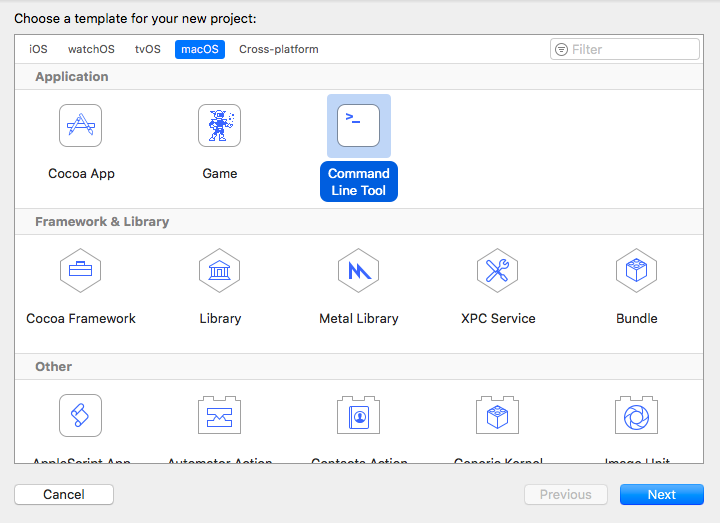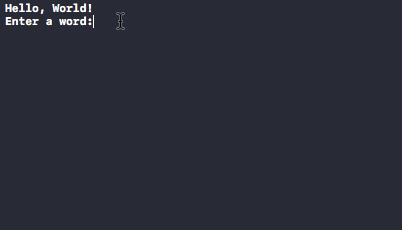#### // Tutorial //

Published on August 3, 2022By Anupam ChughWhile we believe that this content benefits our community, we have not yet thoroughly reviewed it. If you have any suggestions for improvements, please let us know by clicking the “report an issue“ button at the bottom of the tutorial.

In this tutorial, we’ll be discussing how to read the standard input in Swift from the user and the different ways to print the output onto the screen. Swift print() is the standard function to display an output onto the screen. Let’s get started by creating a new command line application project in XCode.## Swift Input

In Swift, the standard input is not possible in Playgrounds. Neither in iOS Application for obvious reasons. Command Line Application is the possible way to read inputs from the user. `readLine()` is used to read the input from the user. It has two forms:

• `readLine()` : The default way.
• `readLine(strippingNewLine: Bool)` : This is default set to true. Swift always assumes that the newline is not a part of the input

`readLine()` function always returns an Optional String by default. Add the following line in your `main.swift` file.

``````let str = readLine() //assume you enter your Name
print(str) //prints Optional(name)
``````

To unwrap the optional we can use the following code:

``````if let str = readLine(){
print(str)
}
``````

### Reading an Int or a Float

To read the input as an Int or a Float, we need to convert the returned String from the input into one of these.

``````if let input = readLine()
{
if let int = Int(input)
{
print("Entered input is \(int) of the type:\(type(of: int))")
}
else{
print("Entered input is \(input) of the type:\(type(of: input))")
}
}
``````

For Float, replace Int initialiser with the Float.

The following code reads multiple inputs and also checks if any of the input is repeated.

``````while let input = readLine() {
guard input != "quit" else {
break
}

if !inputArray.contains(input) {
inputArray.append(input)
print("You entered: \(input)")
} else {
}

print()
print("Enter a word:")
}
``````

The `guard let` statement is used to exit the loop when a particular string is entered `print()` as usual prints the output onto the screen. The current input is checked in the array, if it doesn’t exist it gets added. A sample result of the above code when run on the command line in Xcode is given below.### Reading Input Separated by Spaces

The following code reads the inputs in the form of an array separated by spaces.

``````let array = readLine()?
.split {\$0 == " "}
.map (String.init)

if let stringArray = array {
print(stringArray)
}
``````

split function acts as a delimiter for spaces. It divides the input by spaces and maps each of them as a String. Finally they’re joined in the form of an array.

The following code reads a 2D Array

``````var arr = [[Int]]()
for _ in 0...4 {
var aux = [Int]()

if let x = Int(\$0) {
aux.append(x)
}
else{
print("Invalid")
}
})
arr.append(aux)
}

print(arr)
``````

In the above code we can create 4 sub arrays inside an array. If at any stage we press enter without entering anything in the row, it’ll be treated as a empty subarray.

## Swift print()

We’ve often used `print()` statement in our standard outputs. The print function actually looks like this: `print(_:separator:terminator:)` `print()` statement is followed by a default new line terminator by default

``````print(1...5) //// Prints "1...5"

print(1.0, 2.0, 3.0, 4.0, 5.0) //1.0 2.0 3.0 4.0 5.0

print("1","2","3", separator: ":") //1:2:3

for n in 1...5 {
print(n, terminator: "|")
}
//prints : 1|2|3|4|5|
``````
• terminator adds at the end of each print statement.
• separator adds between the output values.

Concatenating string with values We use `\(value_goes_here)` to add values inside a string.

``````var value = 10
print("Value is \(value)") // Value is 10
``````

This brings an end to this tutorial on Swift Standard Input and Output.

Thanks for learning with the DigitalOcean Community. Check out our offerings for compute, storage, networking, and managed databases.Anupam Chugh

author

#### Still looking for an answer?

Ask a questionSearch for more help

Click below to sign up and get \$200 of credit to try our products over 60 days!

### Popular Topics

##### Join the Tech Talk##### Hollie's Hub for Good

Working on improving health and education, reducing inequality, and spurring economic growth? We’d like to help.